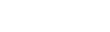# There are many cases where we need to multiply two matrices where one matrix is sparse . Especially in CNN (Convolutional Neural Network) . Lets explore how to do it.Aniruddha Dutta

5 months ago | 2 min read
Follow

In this article we will discuss how to multiply a sparse matrix (particularly in CSR <compressed sparse row> format) with another 2D matrix using cuda .

Why do we need to convert a sparse matrix to CSR format ?🤔

Since sparse matrix mostly contains many zeros , multiplying any element by zero is a redundant operation which consumes processing time. Also if a matrix is sparse we could save our memory by keeping only non zero elements during computation.

How to represent a matrix in CSR format?

We require 3 vectors to do so : Ap , Av , Aj.

Example: Say we have the following matrix A:

Here:
Av = [5 , 8 , 3 , 6] ( contains the non zero elements of matrix ,in left to right and top to bottom fashion )
Aj = [0 , 1 , 2 , 1] ( contains column numbers of elements in Av )
A_temp = [ 0 , 2 , 1 , 1 ] ( A_temp[i] contains number of non zero elements present in ith row )
Ap = [ 0 , 0 , 2 , 3 , 4 ] ( Ap[i] = Ap[i-1] + A_temp[i] , it is prefix sum of A_temp )
NOTE- An extra zero is appended to left of Ap ( line 29 in below code )

## Step1. Let us code a program csr_spmv2.cu to multiply a 1D matrix to our CSR matrix A:

using \$ time ./a.out command we can observe the following output-

real 1m0.762s

user 0m0.076s

sys 0m0.160s

So what does the above output mean ?

For in depth explaination refer this ans Stack Overflow . In short it is taking 1 min 0.762 sec in real time which a lot ! This shows there is significant room for improvement .Lets take a step back and analyse our kernel code. We see that the multiply_row functions output (that is the element of our resultant matrix y ) is calculated by each thread . Which means each thread does 4 multiplication operations in our example ( we have input 4x4 matrix in lines 113 to 128 )

## Step2 . Multiplying CSR matrix with 2D matrix with optimization

Finally we would use a warp to calculate the sum for a row. A warp imples 32 threads will be used to calculate row sum unlike one thread in above code . Also here we would multiply a 2D matrix to our CSR matrix instead of 1D matrix ( For this we would convert the 2D matrix into column major format ).

Is there anything we could do to optimise it further ?

Yes, we could use fast fourier transformation to further increase the speed of computation . However that is beyond the scope of this article .

UpvoteCreated by

Aniruddha Dutta

FollowPost

Upvote

Downvote

Comment

Bookmark

Share

Related Articles